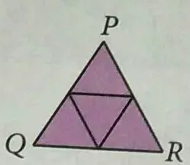# Karim

Karim uses a photocopier to enlarge the triangle PQR diagram by 150%.

a) Write the ratio of the length of P' Q' to the length of PQ.

b) Is the ratio of the length P 'R' to the length PR equal to the ratio of the length P 'Q' to the length PQ?

c) Use your knowledge of ratios and percentages to explain the meaning of ‘enlarging the PQR diagram by 150%’.

Result

r =  1.5
b=

r =

### Step-by-step explanation:Did you find an error or inaccuracy? Feel free to write us. Thank you!

Tips for related online calculators